AP Chemistry : Kinetics and Energy

Example Questions

Example Question #11 : Reaction Rate And Rate Law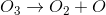(slow)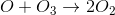(fast)

The mechanism for decomposition of ozone is shown. What is the intermediate of the process?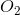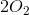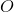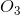Explanation:

Intermediate is created and destroyed, and therefore does not appear on the net equation, which is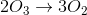. Thus, the intermediate is. Note that when asked for an intermediate, the coefficient in front of it is not used, rather we are looking for the species that is a product of one reaction and a reactant in a subsequent step.

Example Question #18 : Reaction Rate And Rate Law

The rate law of the reaction,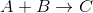, is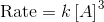. Which of the following does not increase the rate of the reaction?

Increasing the concentration ofAdding the catalyst to the reaction

Increasing the temperature of the reaction

Increasing the concentration ofIncreasing the concentration ofExplanation:

The reactantis not included in the rate law expression, and therefore altering its concentration does not affect the rate of the reaction. Catalysts always increase the rate of reactions by lowering its activation energy. Increasing temperature (average kinetic energy of the molecules) increases the frequency of collisions, and increases the proportion of collisions that have enough energy to overcome the activation energy and undergo a chemical reaction. Increasing the concentration ofwill increase the rate of the reaction as indicated by the rate law.

Example Question #19 : Reaction Rate And Rate Law

Which of the following changes to reaction conditions will always result in an increase in the reaction rate?

Increased concentration of reactants

Increased temperature

Decreased temperature

Decreased concentration of products

Increased temperature

Explanation:

For this question, we're asked to identify something that will always increase the rate of a reaction. Notice that the question specifically states "always."

Let's go through each answer choice and see how it affects the reaction rate.

When the concentration of reactants is increased, this may increase the reaction rate, but not always. For example, if a reaction is zero-order with respect to its reactants, then changing the reactant concentration will have no effect on the rate.

Just like with reactants, decreasing the concentration of products may or may not change the reaction rate. If the reaction rate is zero-order with respect to the products, then a change in their concentration will have no effect on the reaction rate.

A change in temperature is the only thing that is guaranteed to change the reaction rate. This is because changing the temperature will directly change the rate constant of the reaction. Increasing the temperature will increase the rate constant, and hence the reaction rate.

Example Question #2 : Kinetics

The overall reaction can only proceed as quickly as the __________.

rate-determining step

products are removed

intermediate is decomposed

rate-determining step

Explanation:

The rate-determining step in a reaction mechanism is a kinetic bottleneck, in that it prevents the overall reaction from proceeding; thus, it is what determines how quickly the overall reaction can proceed.

Example Question #2 : Principles Of Reaction Kinetics

Which of the following does not affect a forward reaction rate?

Product concentration

Temperature

Catalysts

Medium

Product concentration

Explanation:

Product concentration would not affect a forward reaction rate, since that is what is being formed. Catalysts specifically speed up reaction rates, as does temperature. Medium can also affect reaction rate because some molecules are more likely to react with each other in certain environments.

Example Question #1 : Principles Of Reaction Kinetics

Which of the following is not true of the transition state?

It has a greater energy than the products

Old bonds are weakened and new bonds begin to form

It has a greater energy than the reactants

It cannot dissociate into products without needing any additional energy

It cannot dissociate into products without needing any additional energy

Explanation:

The transition state is the energy barrier in a reaction—energy is needed to reach this state. Once it is acheived, however, it can either revert back to reactants or dissociate into products without any added energy.

Example Question #4 : Principles Of Reaction Kinetics

Which of the following is true:

I. First order reactions proceed at a constant rate

II. First order reactions have a constant half-life

III. First order reactions have one reactant

II and III

I only

I, II, and III

II only

II only

Explanation:

First order reactions have the rate equation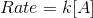. As the molarity of A decreases, the rate slows.

The half-life equation is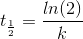. The half-life is constant with respect to time and concentration, depending only on the rate constant.

Lastly, the overall reaction can have any number of reactants. A reaction is first order because its rate-limiting step has only one reactant.

Only statement II is true.

Example Question #5 : Principles Of Reaction Kinetics

The following reversible reaction takes place in a closed container: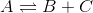The system is allowed to reach equilibrium before 2 moles of reactant A are added to the container.

The net rate of formation of product B immediately following the addition of reactant A is __________.

impossible to determine without additional information

zero

negative

positive

positive

Explanation:

According to Le Chatelier's principle, an increase in the concentration of reactants will cause the reaction to shift to the right in order to re-establish equilibrium. Thus, immediately after reactant A is added, the forward reaction will increase and the net rate of formation of the products will be positive.

Example Question #6 : Principles Of Reaction Kinetics

Rate data is collected for a reaction, and the following integrated rate law is derived.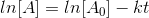If this reaction is __________ with respect to reactant A, a plot of ln[A] versus time would be __________.

second order . . . parabolic

first order . . . parabolic

zero order . . . linear

first order . . . linear

first order . . . linear

Explanation:

The integrated rate law shown is for a reaction rate law with a first-order dependence on reactact A. If such a rate law accurately describes the kinetics of the reaction, then ln[A] will vary linearly with respect to time.

Example Question #1 : Principles Of Reaction Kinetics

Which of the following affects the rate of a reaction?

Frequency of collision

Collision orientation

Molecules' energies

All of these